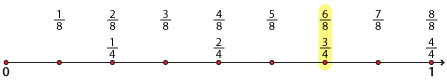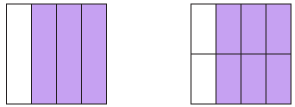#### Example 1

Show that $$\dfrac{3}{4}$$ and $$\dfrac{6}{8}$$ are equivalent fractions using:

1. the number line
2. squares

#### Solution

1. We divide the number line into lengths of $$\dfrac{1}{4}$$ and $$\dfrac{1}{8}$$ and observe that $$\dfrac{3}{4}$$ and $$\dfrac{6}{8}$$ are at the same point on the number line.Detailed description
2. The two equal squares below are divided into four and eight equal parts respectively. We can see that $$\dfrac{3}{4}$$ is the same area as $$\dfrac{6}{8}$$.Detailed description

#### Example 2

Fill in the boxes to complete the equivalent fractions:

1. $$\dfrac{1}{5}=\dfrac{\Box}{20}=\dfrac{\Box}{25}=\dfrac{\Box}{60}$$
2. $$\dfrac{3}{5}=\dfrac{18}{\Box}=\dfrac{\Box}{55}=\dfrac{21}{\Box}$$

#### Solution

1. \begin{align}\dfrac{1}{5}=\dfrac{4}{20}&\hspace{30mm}(5 × 4 = 20\ \text{and}\ 1 × 4 = 4)\\\\ \dfrac{1}{5}=\dfrac{5}{25}&\hspace{30mm}(5 × 5 = 25\ \text{and}\ 1 × 5 = 5)\\\\ \dfrac{1}{5}=\dfrac{12}{60}&\hspace{30mm}(5 × 12 = 60\ \text{and}\ 1 × 12 = 12)\end{align}
2. \begin{align}\dfrac{3}{5}=\dfrac{18}{30}&\hspace{30mm}(3 × 6 = 18\ \text{and}\ 5 × 6 = 30)\\\\ \dfrac{3}{5}=\dfrac{33}{55}&\hspace{30mm}(5 × 11 = 55\ \text{and}\ 3 × 11 = 33)\\\\ \dfrac{3}{5}=\dfrac{21}{35}&\hspace{30mm}(3 × 7 = 21\ \text{and}\ 5 × 7 = 35)\end{align}

#### Example 3

Simplify $$\dfrac{21}{24}$$

#### Solution

$$\dfrac{21}{24}=\dfrac{\color{red}{\setminus}\hspace{-4mm}{21^7}}{\color{red}{\setminus}\hspace{-4mm}{24^8}}=\dfrac{7}{8}$$

So the simplest form is $$\dfrac{7}{8}$$.